# NumXL Cookbook - Volatility Forecast With GARCH

What is the significance of volatility? First, the volatility or standard deviation is an important measure of market risk. Second, it is often used to price derivatives (e.g. options) instruments.

In this paper, we will demonstrate the few steps required to convert the market index S&P 500 data into a robust volatility forecast using the NumXL Add-in within Microsoft Excel

For our purpose, we are using the S&P 500 ETF (aka SPDR) prices as a proxy for the US large-cap equities market. Furthermore, we are using the monthly prices (tabulated at the beginning of the month) ranging from Jan 2000 to Feb 2012.

The objective here is to construct a model-based volatility forecast over the next 12 months (i.e., to the end of Feb. 2013).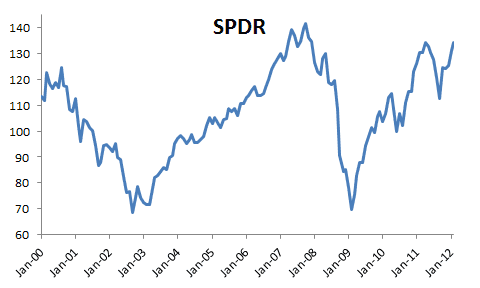### Step 1: Monthly Returns

The time series of the SPDR prices is non-stationary, and, thus is not suitable for many time series or econometric analyses. Therefore, we first converted it to monthly returns. Furthermore, we chose the logarithmic returns over the simple returns to spread out the values of the time series as the simple returns by definition can’t be lower than minus 1 (-100%).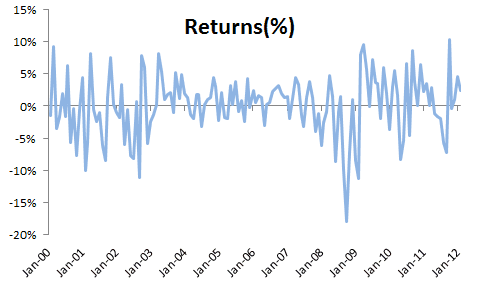In the graph below, we plotted the 12-month weighted moving average (WMA) and the exponential weighted volatility (EWMA) time series to demonstrate the variation of the mean and the volatility over time.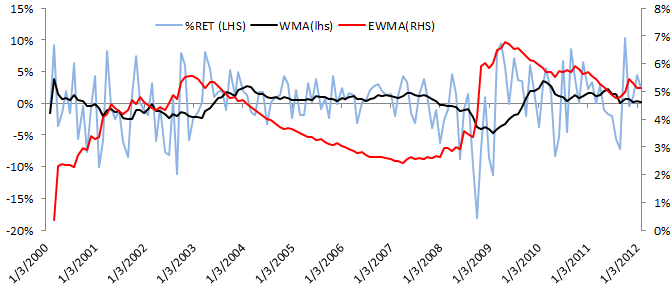Please note that the volatility forecast(proxy by EWMA) moves smoothly (unlike returns), but it is more sensitive to negative returns than it is to a positive returns market.

### Step 2: Summary Statistics

Let’s now calculates the descriptive statistics of the monthly returns sample: average, standard deviation, etc., to help us better understand the data. Built-in functions from NumXL can be utilized as shown to generate a set of statistics to summarize past market trends.

Using the summary statistics wizard, enter the input data set (e.g., returns cells range in column H) into the “Time Series” tab, the starting cell into the output range, and then click OK.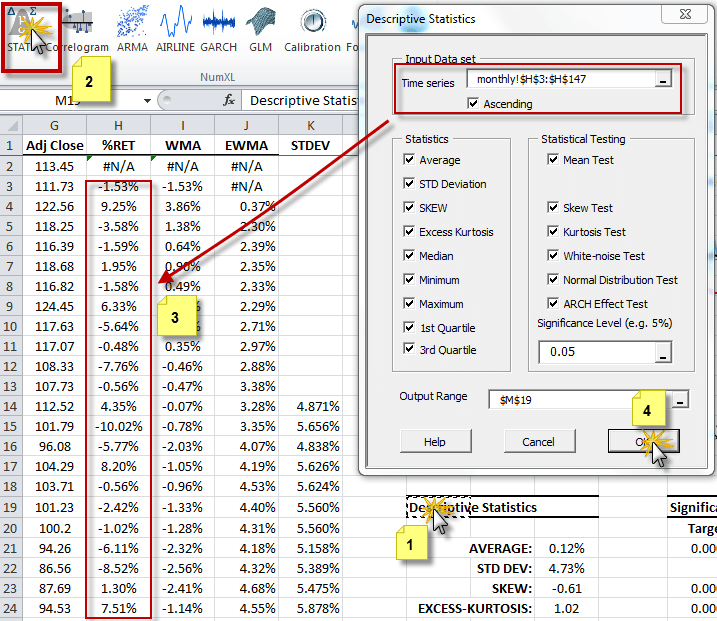The generated output table is shown below. Please note that cells in the output table are connected to the input data sources; the Summary statistics wizard writes the formulas of each output using the labels specified in the first row of each data column.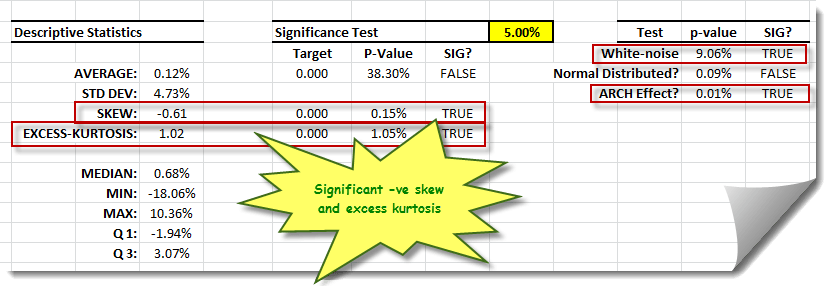Examining the output table demonstrates that the distribution of the log-returns exhibits negative skew (skewed to the left) and fat-tails. Furthermore, the white-noise test result indicates the absence of any significant serial correlation between returns. In sum, these results indicate that these data can be well represented by a GARCH-type model.

### Step 3: E-GARCH Modeling

Early on, we noted that volatility forecast(proxy EWMA) reacted differently to negative returns (downturn) than to positive ones. Fortunately, the exponential GARCH (E-GARCH) captures this phenomenon

NumXL supports three (3) types of distributions for the residuals: (1) Gaussian, (2) Generalized Error Distribution (GED), and (3) Student’s t-distribution. The sample data exhibits relatively low excess kurtosis, so the GARCH model will capture the entire excess kurtosis, thus, permitting the residuals to be normally distributed (i.e. Gaussian).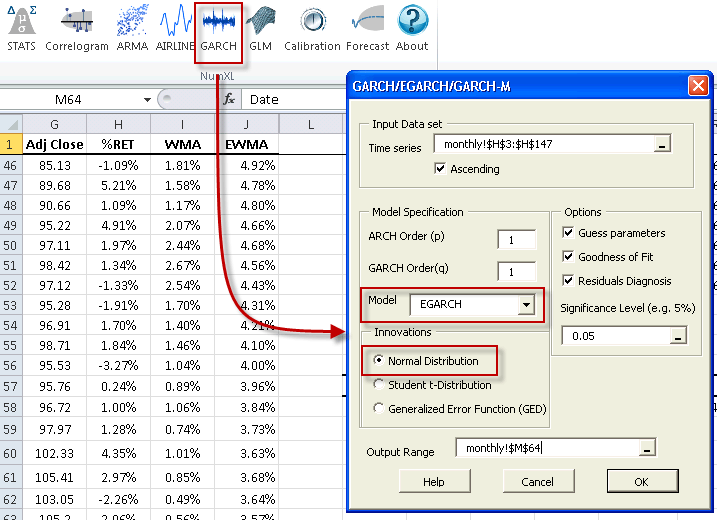After entering the input data set into Time Series and the output range cell, the model can be selected and must be primed by entering some model-specific parameters. Please note that while these values are not yet known, a crude and intelligent guess should be entered.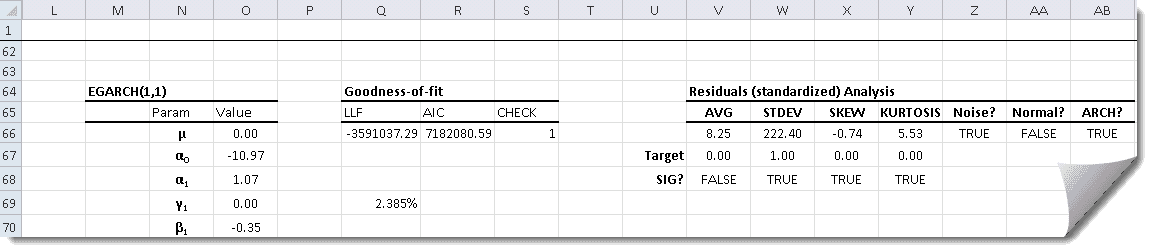As in the summary statistics, the cells in the E-GARCH output table are connected to the source input data via the formulas.

### Step 4: E-GARCH Calibration

To fit (i.e. calibrate) the model with our sample data: (1) select the cell labeled “EGARCH(1,1)”, (2) click on the Calibrate icon or menu item, and finally, (3) click on the Solve button.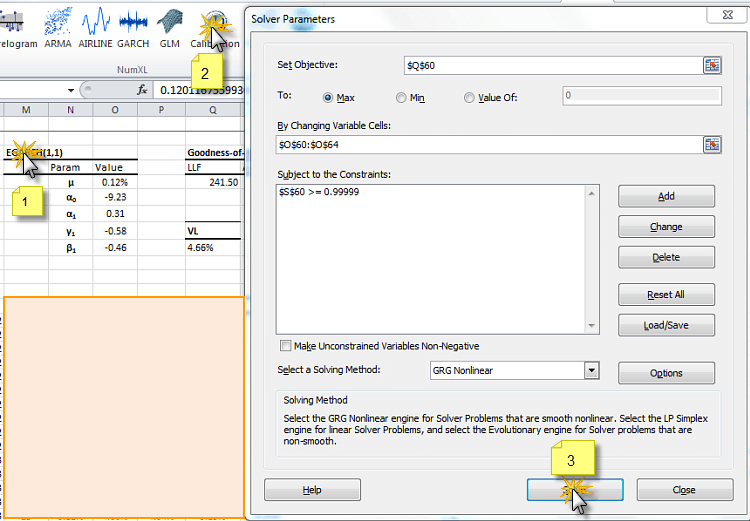The MS Excel Solver will maximize the log-likelihood function (LLF) by altering the values of the coefficients.

### Step 5: Residual Diagnosis

Once the E-GARCH model’s coefficients are calibrated, we can examine the model’s standardized residuals to make sure that they satisfy the underlying assumptions of the model (i.e., normally distributed).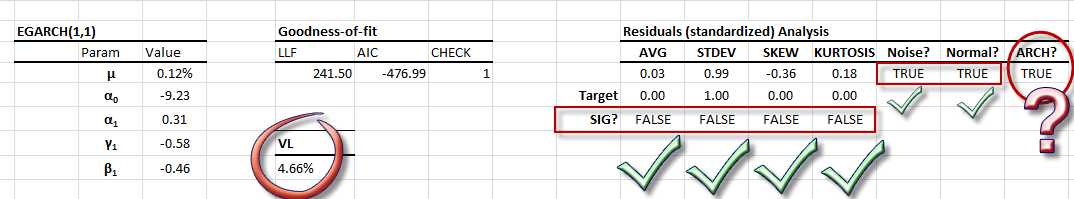Using the residuals diagnosis table, we note all tests pass with the mere exception of the ARCH test which suggests the presence of a higher-order (i.e. quadratic) dependency. For the purpose of this paper, we will accept the calibrated model.

The GARCH family of models captures a common and important phenomenon for volatility: mean-reversion. Using our E-GARCH model, the long-term monthly volatility is estimated at 4.66% (or 16.14% annually).

### Step 6: Volatility Forecast

The GARCH-family of models describes the variation of one-step (i.e., local) volatility over time, but, in practice, we need volatility values that span multi-steps (i.e., global or term). In this paper, we will prepare both the local and the term volatilities over the next 12 months.

To accomplish this, (1) select the cell with “EGARCH(1,1)” Text, (2) click on “Forecast” icon or menu, select the latest (3) realized returns and (4) volatilities, (5) change the forecast horizon and (6) specify the output location. Finally, select “OK.”.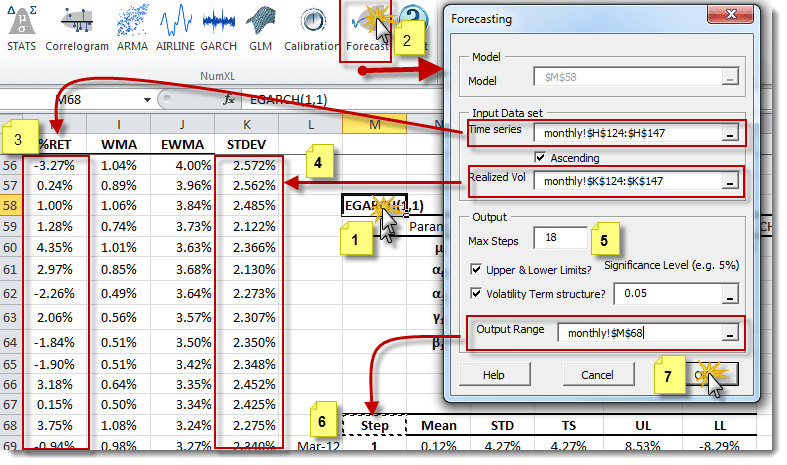Notes

1. 1. The input data should represent the most recent observations. For the E-GARCH (1, 1) model, at least one or two observed returns are required.
2. 2. The Realized volatility forecast(input data) is the most recent volatility. Since volatility is not directly observed, you would need to compute it using your favorite method. In this example, the 12-month window standard deviation was used.

The table output by the NumXL forecast is: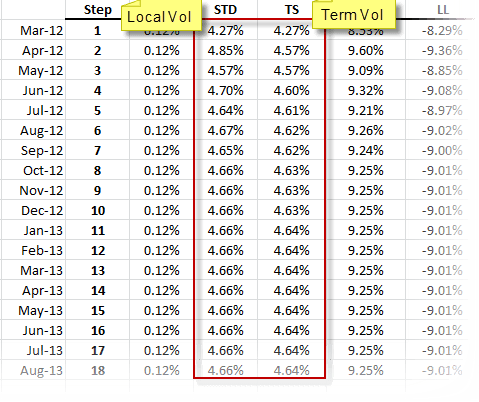The E- GARCH model states that we are currently in a historically low-volatility arena, and it forecasts a rise (mean reversion) in the overall volatility to its long-run level (4.66% /mo. Or 16.14%/yr.).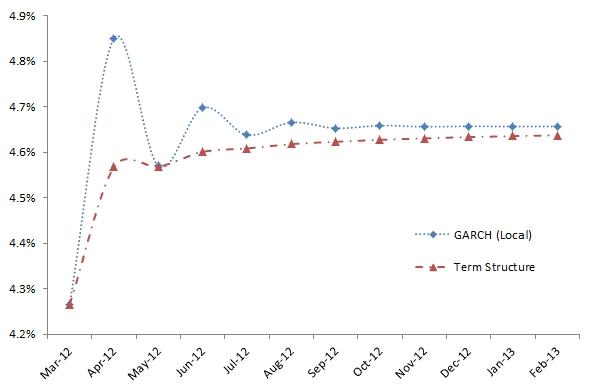More specifically, these results indicate that for the month of February -2012 (i.e., ending March 1st, 2012), we forecast a lower volatility than Jan 2012 because the value is less than the 4.66% long-run baseline However, this volatility is expected to increase in March as it reverts to its long-term mean of 4.66%.